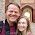Tuesday, March 26, 2013

A simple simulation of Hierarchical Linear Modelling (HLM) using two different R packages - intercept only RE

# HLM is a common tool used to analysize hierarchically structured data.

# Let's see it in action using a couple of the tools programmed up by HLM researchers.

# First let's generate a data set.

# Imagine we have a two level model, students nested within classrooms.

# Let's say we have 20 classrooms

nclass = 20

# And thirty students per classroom

nstud = 30

# Let's imagine that we have a classroom effect that varies randomly and is uncorrelated with the student level effect.

class.effect = rnorm(nclass)*2

# Imagine also we have a unique outcome per student.

# We have nclass*nstud number of students in total.

student.effect = rnorm(nstud*nclass)*3

# Now we have all the data we need in order to generate our analysis.
data.set = data.frame(class.id = rep(1:nclass, each=nstud),
class.effect = rep(class.effect, each=nstud),
student.id = 1:(nstud*nclass),
student.effect = student.effect)

data.set\$outcomes = data.set\$class.effect + data.set\$student.effect

# Looking good.  Now let's load our HLM package.

require(nlme)

# For quick reference I used:
# https://txcdk.unt.edu/iralab/sites/default/files/HLM_R_Handout.pdf

lme(outcomes ~ 1, random = ~ 1 | class.id, data=data.set)

# We can see that lme is pretty good at estimating a standard deviation of the level 2 effect at around 2 and a level 3 effect around 3.

# Alternatively we can attempt to use the software lme4.
require(lme4)

# For quick reference I went to:
http://lme4.r-forge.r-project.org/book/Ch1.pdf

lmer(outcomes ~ 1 + (1|class.id), data=data.set)
# Reassuringly we can see that the estimates are indentical though lme4 seems to provide more better organized feedback.

1.As you show, it's relatively straightforward to simulate independent data. But what about a situation where student and class are not independent of each other? Taking it to an even more complex level, what about simulating student-level and classroom-level covariates that are perhaps intercorrelated with other predictors and with the outcome in a regression model? It would be interesting to learn how to simulate data with dependencies such as these. Does R provide an easy way to create such simulations?

1.This is a good point. Independence is a large assumption that should not be lightly made. I will post more on this is the future.

2.Dear Dr. Smart,

I have a similar data analyzes challenge (ecological data) but maybe with a little more complexity. I have 27 sites, each with 3 plots (27 x 3 = 81 rows in my data). So plots are nested within sites. I want to analyze the effect of 3 environmental variables on the abundance of insects found in each of those plots/sites. So lets call those variables X1, X2, X3. At least two of the 3 environmental variables (X2, X3) was taken at plot level so it can differentiate between plots within the same site. The other environmental were taken at site level so they have the same value for the 3 plots within a site. This is just a description about why I have been using mixed models.

The tricky part - which is the reason of my post - is the way to enter X3 in the analyzes, which is an index describing the landscape 2000 meters around each site. Previously I have been entering it just as a mean value for every site. That is, I took the mean of all the pixel values 2000 meters around each site. That's pretty straigth forward. However I think using a mean is a pretty coarse measure. Instead of that I would like to weigth the values of all the pixels by its distance to the site. To do that I want the model to estimate a parameter for that term during the weighting process. In other words, my model was:

Y = b1.X1 + b2.X2 + b3.X3,

where b's are the parameters estimated for the model and X3 was a mean of Lanscape around

Now I want,

Y = b1.X1 + b2.X2 + exp((Dist/b3)*X3),

where X3 and Dist are the pixel value and its distance (for all the 14.000 pixels around each plot).

In few words, in order the model to run, it has to analyze the values of Y, X1 and X2 from every line in data set #1 (81 rows = 81 plots), and for each of those 81 rows, to analyze X3, it has to go to the data set #2 (1'134.000 rows) and analyze the rows that correspond to the particular plot (row) in data set #1.

I know the situation could sounds complicated, but is not... if I was clear enough. What could be complicated is the way to analyze the data in the way I explained. Any advice about how to run this analyzis, potential R packages to use, will be appreciated. I thionk it could be done with Maxim-Likelihhod or maybe better with MCMC... but don't know exactly how to implement that in R.